Updated by Laramy Wells on May 01, 2015
REPORTLaramy Wells
Owner
8 items   1 followers   1 votes   5 views

# Trigging Around the Map

The following resources will assist you with the Trigging Around the Map activity. You will find resources regarding finding distance with latitude and longitude as well as information regarding the Law of Cosines, the Law of Sines, and Area. When you find the most useful resource be sure to "like" it so that other students can be directed to the best resources.

3

## Calculate distance and bearing between two Latitude/Longitude points using haversine formula in JavaScript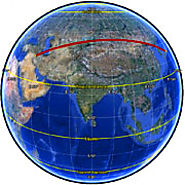This page presents a variety of calculations for latitude/longitude points, with the formulæ and code fragments for implementing them. All these formulæ are for calculations on the basis of a spherical earth (ignoring ellipsoidal effects) - which is accurate enough* for most purposes...

1

## Latitude/Longitude Distance Calculator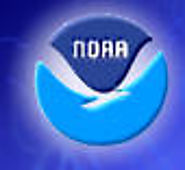The distance calculator on this page is provided for informational purposes only. The calculations are approximate in nature and may differ a little from the distances as given in the official forecasts and advisories.

2

## Finding distances based on Latitude and Longitude.

This formula does not take into account the non-spheroidal (ellipsoidal) shape of the Earth. It will tend to overestimate trans-polar distances and underestimate trans-equatorial distances. The values used for the radius of the Earth (3961 miles & 6373 km) are optimized for locations around 39 degrees from the equator (roughly the Latitude of Washington, DC, USA)

4

## Latitude/Longitude Distance Calculation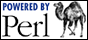This calculation considers that the Earth is a perfect sphere.

5

## Law of Cosines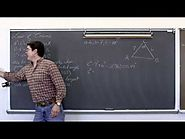I introduce and do examples of solving oblique triangles using the law of cosine. Check out http://www.ProfRobBob.com, there you will find my lessons organized by class/subject and then by topics within each class.

6

## Ambiguous Case for Law of Sines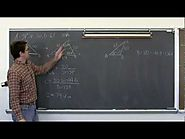I introduce solving oblique triangles when the information given is in the SSA form...or the ambiguous case. In this setting there may be one solution, two solutions, or none at all. NOTE: At minute 14:37 I incorrectly stated 61*sin(39)=37.8, it is 38.4. At minute 16:50 I state that angle B is 48.4 degrees.

7

## Area of oblique triangles SAS SSS Heron's Formula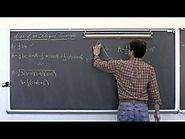I introduce and do examples of finding the area of oblique triangles using two sides and an includeds angle, as well as using Heron's formula when you know all three sides. Check out http://www.ProfRobBob.com, there you will find my lessons organized by class/subject and then by topics within each class.

8

## Covington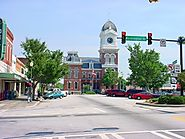Be reminded that one of your points MUST be from Covington, Georgia. The latitude and longitude of Covington is: 33.5968° N, 83.8616° W